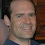## Wednesday, June 10, 2009

### Can we make this problem FUN?

Consider the following cute problem:
Alice, Bob, and Carol each have a number between 0 and 1,000,000,000,000 on their forehead. Everyone can see the numbers that are NOT on their own forehead. Call the three numbers x,y,z. They wish to determine if x+y+z is divisible by 1,000,000,000,000. There is an easy solution: Alice can just say Bob, your number is .... This would take 13 digits of communication. Can they do better?
Chandra, Furst, and Lipton showed that if instead of 1,000,000,000,000 was replaced by 2n, and if n is large, then do this in roughly \sqrt n bits. They were more interested in the k-party lower bound of &omega(1) since this leads to lower bounds on branching programs. (NOTE: They studied a variant of the the problem above, but everything they did applies hear also.) See their paper or my writeup for JUST the upper bound or my writeup of the upper and lower boundand its application to branching programs for more information.

The problem stated above with people having numbers on their foreheads sounds like it could be a fun problem for the layperson. BUT: The problem with this problem for the layperson is two fold: (1) The original problem involves 3-free sets. While our community might say Wow, an application of stuff coming out of van der Waerden's theorem (or at least I would say that), the layperson would not think of it or care. (2) Frankly, I don't know if 13 digits numbers are big enough for the asymptotics to kick in and give a better answer than 13.

SO- here is my challenge: Is there a solution to this problem (or perhaps one with larger numbers) where the solution is non-trivial--- that is, you do not communicate all of the numbers, but is also FUN! FUN means you can tell it to your non-mathematical-nephew, or perhaps your 10-years-old-great-niece-who-likes-math. Quite okay if its far worse than \sqrt(n).

1.some slight error in the statement - you either mean \sqrt{log n} or "10^13 was replaced by an n-bit number"...

2.Fixed- 10^13 is replaced by 2^n

bill g.

3.Supposedly, you are looking for a deterministic solution? If an error probability is allowed, one can employ the protocol for 2-party equality testing (twice).

4.One can do n/2 bits (or digits for your ten fingered friends). Basically have one player say the XOR of the first digit of player 1 with the last digit of player 2. Repeat for the second and the pentultimate digit. Once you have gone 1/2 way through, one of the other two players now knows if there is an error. If they both haven't found an error, then the 3 numbers add up as desired. So this is a n/2 + 2 communications. Way off of sqrt(n), but at least much better than n itself.

5.1 trillion in base 2 has many more digits than 1 trillion in base 10. If you did it in base 1-trillion - each number would be a single symbol.

You could tell an approximate square root for about 6 digits in base 10. An approximate log base-10 for even fewer digits.

What you are doing is encoding some of the information of the message in an externally agreed upon set of operations. There is a second conduit of information: the communication protocol itself.